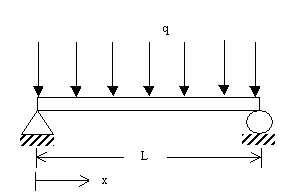# Quiz Chapter 08.07: Finite Difference Method

 MULTIPLE CHOICE TEST FINITE DIFFERENCE METHOD ORDINARY DIFFERENTIAL EQUATIONS

1. The exact solution to the boundary value problem

• $\dfrac{d^{2}y}{dx^{2}} = 6x - 0.5x^{2}, \, y(0) = 0, \, y(12) = 0$

for $y(4)$ is

2. Given

• $\dfrac{d^{2}y}{dx^{2}} = 6x - 0.5x^{2}, \, y(0) = 0, \, y(12) = 0$

the value of $\dfrac{d^{2}y}{dx^{2}}$ at $y(4)$ using the Finite Difference Method and a step size of $h=4$ can be approximated by

3. Given

• $\dfrac{d^{2}y}{dx^{2}} = 6x - 0.5x^{2}, \, y(0) = 0, \, y(12) = 0$

The value of $y(4)$ using the Finite Difference Method with a second order accurate central divided difference method and a step size of $h=4$ is

4. The transverse deflection, $u$ of a cable of length, $L$, fixed at bothe ends is given as a solution to

• $\dfrac{d^{2}u}{dx^{2}} = \dfrac{Tu}{R} + \dfrac{qx \left( x - L \right)}{2R}$

where

• $T=$ tension in cable
• $R=$ flexural stiffness
• $q=$ distributed transverse loadGiven values are $L=50", \, T=2000$lbs, $q=75$ lbs/in, $R=75 \times 10^{6}$ lbs⋅in2, using Finite Difference Method modeling with second order central divided difference accuracy and a step size of $h=12.5"$, the value of the deflection in inches at the center of the cable is most nearly

5. The radial displacement, $u$ is a pressurized hollow, thick cylinder (inner radius$=5"$, outer radius$=8"$) is given at different radial locations.

 Radius (in.) Radial Displacement (in.) $5.0$ $0.0038731$ $5.6$ $0.0036165$ $6.2$ $0.0034222$ $6.8$ $0.0032743$ $7.4$ $0.0031618$ $8.0$ $0.0030769$

The maximum normal stress, in psi, on the cylinder is given by

• $\sigma_{max} = 3.2967 \times 10^{6} \left( \dfrac{u(5)}{5} + 0.3 \dfrac{du}{dr}(5) \right)$

The maximum stress, in psi, with second order accuracy is

Hint: $f(x_{i+1}) = f(x_{i}) + {f}'(x_{i})h + \dfrac{{f}''(x_{i})h^{2}}{2} + \dfrac{{f}'''(x_{i})h^{3}}{6} + \, ... \,$,

and $f(x_{i+2}) = f(x_{i}) + {f}'(x_{i})(2h) + \dfrac{{f}''(x_{i})(2h)^{2}}{2} + \dfrac{{f}'''(x_{i})(2h)^{3}}{6} + \, ... \,$

where $x_{i+1} = x_{i} + h$

6. For a simply supported beam (at $x=0$ and $x=L$) with a uniform load $q$, the deflection $v(x)$ is described by the boundary value ordinary differential equation as

• $\dfrac{d^{2}v}{dx^{2}} = \dfrac{qx(L-x)^{2}}{2EI}, \, 0 \leq x \leq L$

where

• $E=$ Young’s modulus of elasticity of beam
• $I=$ second moment of cross-sectional areaThis is based on assuming that $\dfrac{dv}{dx}$ is small; if $\dfrac{dv}{dx}$ is not small, then the ordinary differential equation is